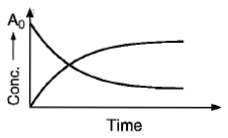# NEET Chemistry Chemical Kinetics Questions Solved

For the reaction , at the point of intersection of two curves show, the [B] is can be given by:(a) $\frac{n{A}_{0}}{2}$

(b) $\frac{{A}_{0}}{n-1}$

(c) $\frac{n{A}_{0}}{n+1}$

(d) $\left[\frac{n-1}{n+1}\right]{A}_{0}$